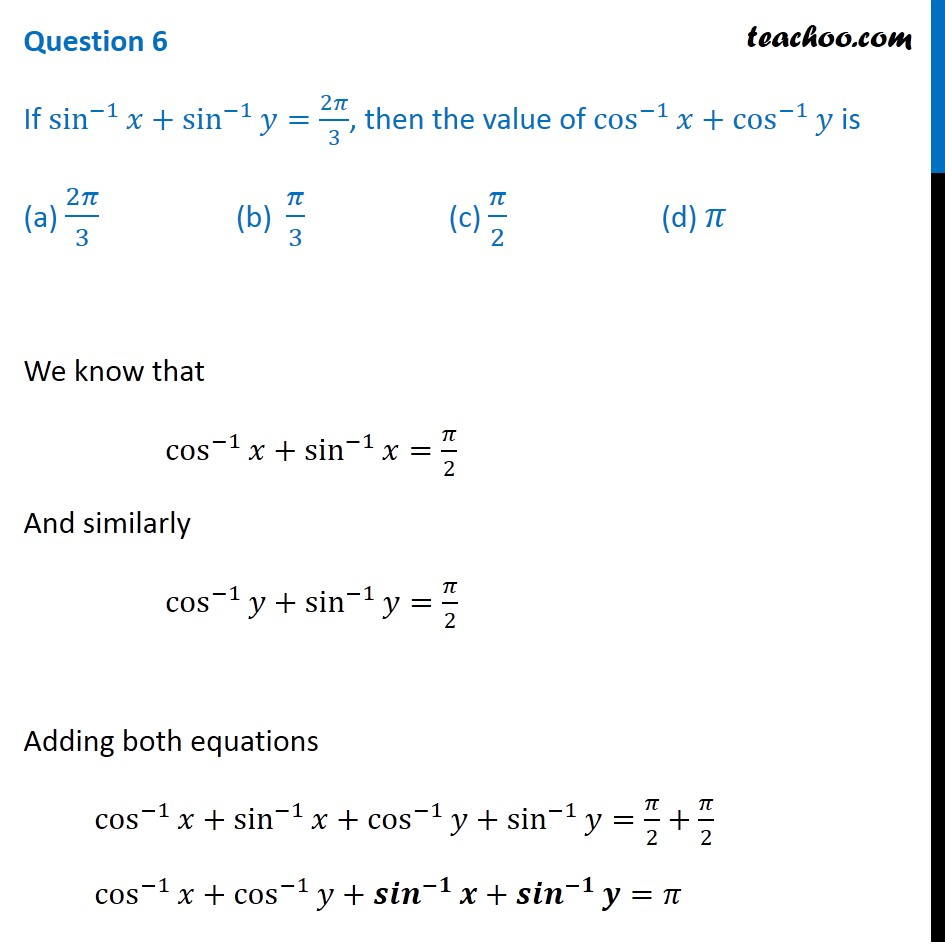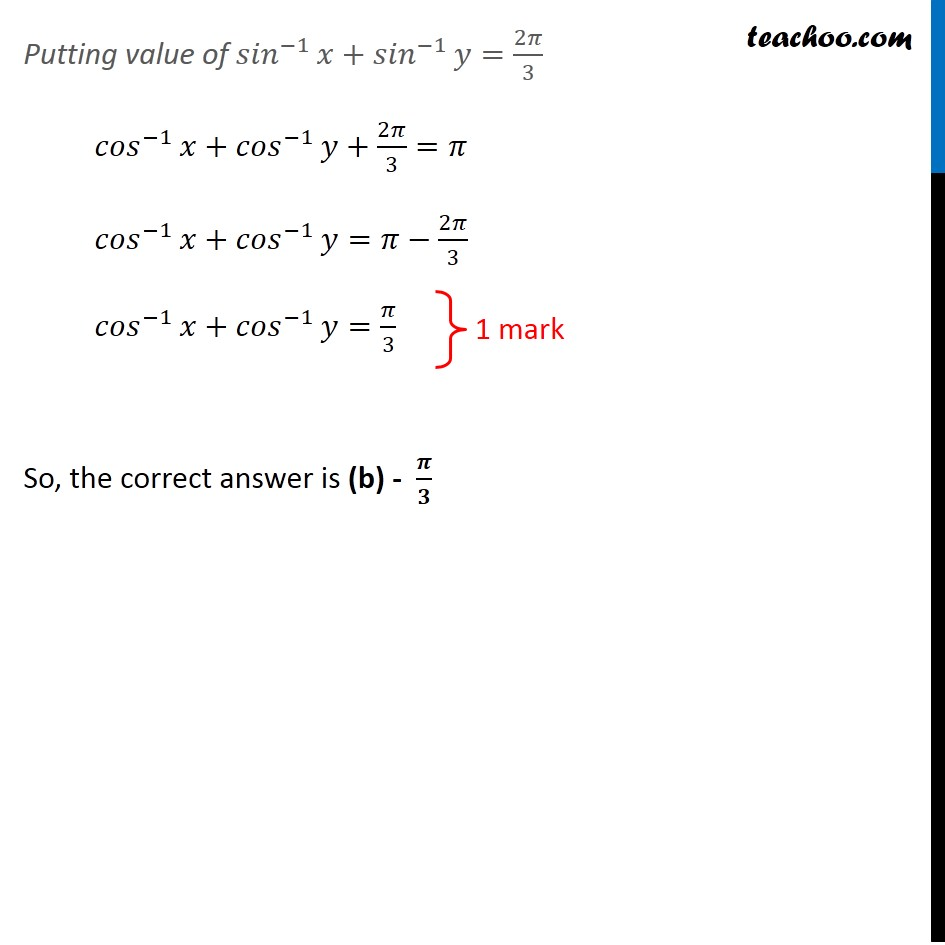## (a) 2π/3                 (b)  π/3              (c) π/2              (d) πSubscribe to our Youtube Channel - https://you.tube/teachoo

1. Class 12
2. Solutions of Sample Papers and Past Year Papers - for Class 12 Boards
3. CBSE Class 12 Sample Paper for 2020 Boards

Transcript

Question 6 If sin^(−1)⁡𝑥+sin^(−1)⁡𝑦=2𝜋/3, then the value of cos^(−1)⁡𝑥+cos^(−1)⁡𝑦 is (a) 2𝜋/3 (b) 𝜋/3 (c) 𝜋/2 (d) 𝜋 We know that cos^(−1)⁡𝑥+sin^(−1)⁡𝑥=𝜋/2 And similarly cos^(−1)⁡𝑦+sin^(−1)⁡𝑦=𝜋/2 Adding both equations cos^(−1)⁡𝑥+sin^(−1)⁡𝑥+cos^(−1)⁡𝑦+sin^(−1)⁡𝑦=𝜋/2+𝜋/2 cos^(−1)⁡𝑥+cos^(−1)⁡𝑦+〖𝒔𝒊𝒏〗^(−𝟏)⁡𝒙+〖𝒔𝒊𝒏〗^(−𝟏)⁡𝒚=𝜋 Putting value of 〖𝑠𝑖𝑛〗^(−1)⁡𝑥+〖𝑠𝑖𝑛〗^(−1)⁡𝑦=2𝜋/3 〖𝑐𝑜𝑠〗^(−1)⁡𝑥+〖𝑐𝑜𝑠〗^(−1)⁡𝑦+2𝜋/3=𝜋 〖𝑐𝑜𝑠〗^(−1)⁡𝑥+〖𝑐𝑜𝑠〗^(−1)⁡𝑦=𝜋−2𝜋/3 〖𝑐𝑜𝑠〗^(−1)⁡𝑥+〖𝑐𝑜𝑠〗^(−1)⁡𝑦=𝜋/3 So, the correct answer is (b) - 𝝅/𝟑 1 mark

CBSE Class 12 Sample Paper for 2020 Boards

Class 12
Solutions of Sample Papers and Past Year Papers - for Class 12 Boards